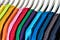# First Things First —What are Data Structures and Algorithms?

Computer science was developed as a way of leveraging computers to solve mathematical problems quickly. In a general computer science problem, you take some data as an input and return some data as an output.

The series of computational steps you need to take to turn that input data into an output data is called an algorithm, and the way the data is organized has a direct impact on the efficiency of the algorithm. A data structure is a way to store and organize data so that it can be used efficiently. Using algorithms, we can perform operations to modify or access data, referred to as elements, in a data structure. Depending on the purpose, each data structure has its own strengths and constraints.

Let’s picture this.Image from Pixabay

Think of your closet as a data structure, and the clothing items in it as the data (a.k.a the elements.) Let’s say you want to access a pair of jeans from the top shelf of your closet. First, if you’re short like myself, you will probably grab a stool to help reach the top shelf. Next, you will go through the stack of jeans until you reach the pair you’re looking for. You can probably try different variations of steps, or algorithms, to get your jeans. For example, you can try moving your stack of jeans to the bottom self and sorting the stack of jeans by wash so that you can access your favorite pair easily going forward.

However, at the end of the day, these variations of steps will be limited to the functionalities and the structure of your closet. A clothing store, for example, will need a more sophisticated closet because the clothing item the sales associates will need to access will vary by the hour and it would be inefficient to keep moving things around. Therefore, a clothing store should consider opting for a rotating closet with waterfall hangers instead of a regular closet.

Four basic operations that most data structures support are: search (check whether an element is contained in a data structure or not, if yes, return it), access (if an element exists in a data structure and you know where it is, return it), insert and delete.

There are different types of data structures, for example: arrays, linked lists, stacks, queues, trees, ... (We’ll go over each of them in detail in the following weeks.) As noted earlier, the choice of data structure has a direct impact on the efficiency of an algorithm. To understand why that’s the case, we need to take a closer look at what it means for an algorithm to be efficient.

In general, you can measure the efficiency of an algorithm by examining its two components: “time complexity” and “memory complexity”. For sake of simplicity, we will only cover time complexity for now. The time complexity of the algorithm is the relation between the number of steps an algorithm will have to complete and the size of the input. You would use Big O notation to describe this complexity in algebraic terms.

Let’s go back to the jeans. Say you work at a store where you receive a stack of jeans in random order from the warehouse daily, and you have to sort them by color. The number of jeans that you have to process daily is called an “input size.” Being the employee of the month, let’s say you have a well established way of tackling this task, and you want to figure out the time complexity to see how efficient it is. If sorting 4 jeans takes 8 steps, sorting 100 jeans takes 664 steps and sorting 1,000 jeans takes 9965 steps, you can see that the relation between the input size and the number of steps is n*log(n), where n is the size of the input (i.e. the number of jeans). You can then say that the time complexity of your way of sorting the jeans (a.k.a your algorithm) is O(n*log(n)) in Big O notation.

Going back to data structures — how can the choice of data structures influence the time complexity of an algorithm? The answer is simple: remember the 4 basic operations we mentioned above? Each data structure will have a different time complexity for search, access, insert and delete. For example, assuming you keep a pointer to the tail, adding an element to the end of a linked list is a O(1) [i.e. constant time] operation, while adding to an array is O(n) [because you might have to copy all elements to a bigger array if you run out of space]. Depending on what operations an algorithm uses and on what data structure, its time complexity will be different. This is why it’s crucial to choose the right data structure for the right problem.

There’s more to unpack on the topic, and we’ll do so each week in the following articles. In the meantime, drop any questions or comments you have below.

## More from Fullstack Moon

all things full stack development.